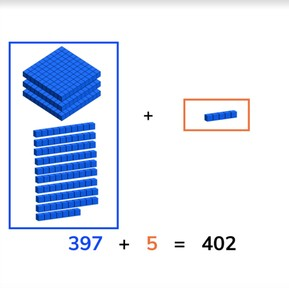Addition to 1,000 with crossing tens and hundreds

# Addition to 1,000 with crossing tens and hundreds

Students learn to add numbers to 1,000 crossing tens and hundreds.8,000 schools use Gynzy92,000 teachers use Gynzy1,600,000 students use Gynzy

## General

Students learn to add numbers to 1,000 crossing tens and hundreds.

2.NBT.B.7

## Relevance

Discuss with students that it is important to be able to add numbers to 1,000 crossing tens and hundreds so you can determine total quantities.

## Introduction

Show a few math problems on the interactive whiteboard and ask students to determine which student has the correct answer. Have them explain their thinking.

## Development

Check that students are able to add numbers to 1,000 crossing tens and hundreds by asking the following questions:
- Why is it useful to be able to add numbers to 1,000?
- Which methods can you use to add the numbers in an addition problem?

## Guided practice

Students are given addition problems with visual support, without visual support, and in story form.

## Closing

Discuss with students that it is important to be able to add numbers to 1,000 crossing tens and hundreds, so you are able to determine the total quantity you have. Show the money on the interactive whiteboard and ask which math problem represents the money shown. Then show a problem for students to solve and discuss their answers. Next solve a story problem as a class. Make sure to discuss the steps of a story problem. Have each students write a number between 1 and 500 on a sheet of paper. Have them form pairs and then solve the addition problem that coms from adding their two numbers together.

## Teaching tips

Students who have difficulty with this learning goal can be supported by making use of manipulatives like MAB blocks or a number line. Remind them that they can make jumps of any size on the number line. Start by having students practice adding numbers that cross tens, then those that cross hundreds. By starting with easier problems like 294 + 9 and increasing the difficulty, you build their confidence.

## Instruction materials

Paper
Optional: MAB blocks or other manipulatives

### The online teaching platform for interactive whiteboards and displays in schools

• Save time building lessons

• Manage the classroom more efficiently

• Increase student engagement